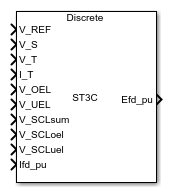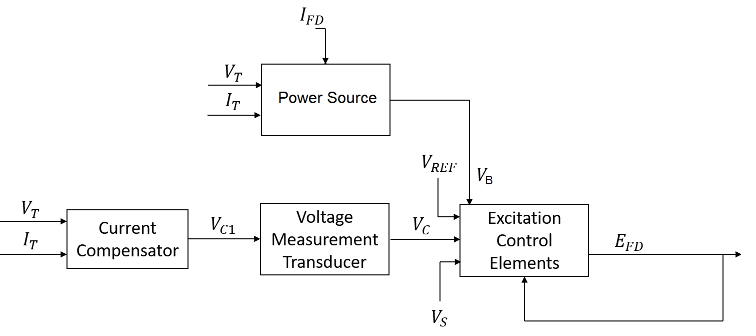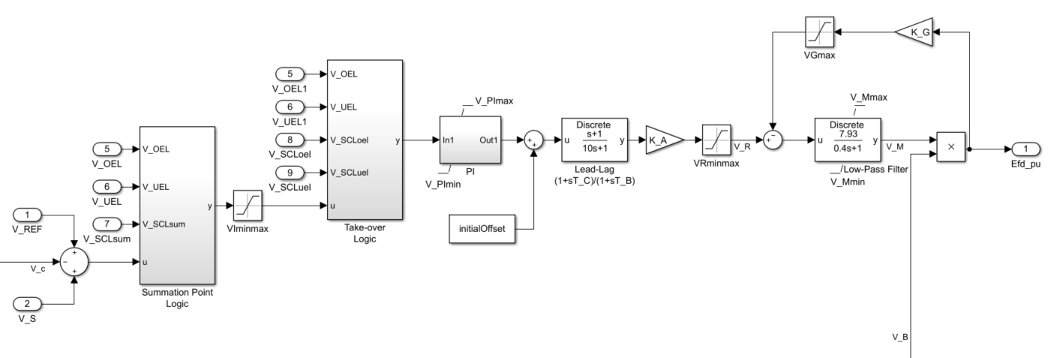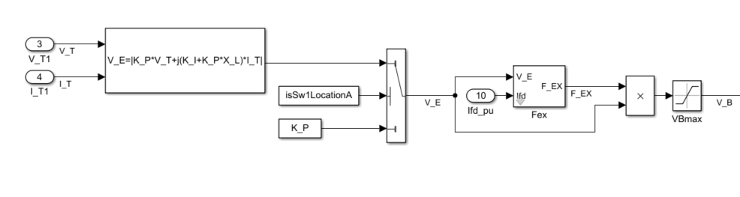# SM ST3C

Discrete-time or continuous-time synchronous machine ST3C static excitation system with an automatic voltage regulator

• Library:
• Simscape / Electrical / Control / SM Control

•## Description

The SM ST3C block implements a synchronous-machine-type ST3C static excitation system model in conformance with IEEE 421.5-2016.

Use this block to model the control and regulation of the field voltage of a synchronous machine.

You can switch between continuous and discrete implementations of the block by using the Sample time (-1 for inherited) parameter. To configure the integrator for continuous time, set the Sample time (-1 for inherited) property to `0`. To configure the integrator for discrete time, set the Sample time (-1 for inherited) property to a positive, nonzero value, or to `-1` to inherit the sample time from an upstream block.

The SM ST3C block comprises four major components:

• The Current Compensator modifies the measured terminal voltage as a function of the terminal current.

• The Voltage Measurement Transducer simulates the dynamics of a terminal voltage transducer using a low-pass filter.

• The Excitation Control Elements component compares the voltage transducer output with a terminal voltage reference to produce a voltage error. This voltage error is then passed through a voltage regulator to produce the field voltage.

• The Power Source models the power source for the controlled rectifier when it is independent from the terminal voltage.

This diagram shows the overall structure of the ST3C excitation system model:In the diagram:

• VT and IT are the measured terminal voltage and current of the synchronous machine.

• VC1 is the current-compensated terminal voltage.

• VC is the filtered, current-compensated terminal voltage.

• VREF is the reference terminal voltage.

• VS is the power system stabilizer voltage.

• VB is the exciter field voltage.

• EFD and IFD are the field voltage and current, respectively.

The following sections describe each of the major parts of the block in detail.

### Current Compensator and Voltage Measurement Transducer

The current compensator is modeled as:

`${V}_{C1}={V}_{T}+{I}_{T}\sqrt{{R}_{C}^{2}+{X}_{C}^{2}},$`

where:

• RC is the load compensation resistance.

• XC is the load compensation reactance.

The voltage measurement transducer is implemented as a Low-Pass Filter block with the time constant TR. Refer to the documentation for the Low-Pass Filter block for the discrete and continuous implementations.

### Excitation Control Elements

This diagram illustrates the overall structure of the excitation control elements:In the diagram:

• The Summation Point Logic subsystem models the summation point input location for the overexcitation limiter (OEL), underexcitation limiter (UEL), stator current limiter (SCL), and the power switch selector (V_S) voltages. For more information about using limiters with this block, see Field Current Limiters.

• The Take-over Logic subsystem models the take-over point input location for the OEL, UEL, and SCL voltages. For more information about using limiters with this block, see Field Current Limiters.

• The PI subsystem models a PI controller that functions as a control structure for the automatic voltage regulator and allows the representation of an equipment retrofit with a modern digital controller. The minimum and maximum anti-windup saturation limits for the block are VPImin and VPImax, respectively.

• The Lead-Lag block models additional dynamics associated with the voltage regulator and represents the system stabilizer. Here, TC is the lead time constant and TB is the lag time constant. Refer to the documentation for this block for the exact discrete and continuous implementations.

• An inner field voltage control loop is utilized to linearize the exciter control characteristic and it is composed of the gains KM and KG and the time constant TM. The minimum and maximum anti-windup saturation limits for the block are VMmin and VMmax, respectively.

### Field Current Limiters

You can use various field current limiters to modify the output of the voltage regulator under unsafe operating conditions:

• Use an overexcitation limiter to prevent overheating of the field winding due to excessive field current demand.

• Use an underexcitation limiter to boost field excitation when it is too low, which risks desynchronization.

• Use a stator current limiter to prevent overheating of the stator windings due to excessive current.

Attach the output of any of these limiters at one of these points:

• The summation point as part of the automatic voltage regulator (AVR) feedback loop

• The take-over point to override the usual behavior of the AVR

If you are using the stator current limiter at the summation point, use the single input VSCLsum. If you are using the stator current limiter at the take-over point, use both the overexcitation input, VSCLoel, and the underexcitation input, VSCLuel.

### Power Source

It is possible to adopt a different representation of the power source for the controlled rectifier by selecting the relevant option in the Power source selector parameter. The power source for the controlled rectifier can be either derived from the terminal voltage (```Position A: power source derived from generator terminal voltage```) or it can be independent of the terminal voltage (```Position B: power source independent of generator terminal conditions```).

This diagram shows a model of the exciter power source utilizing a phasor combination of the terminal voltage, VT, and terminal current, IT:## Ports

### Input

expand all

Voltage regulator reference set point, in per-unit representation, specified as a scalar.

Data Types: `single` | `double`

Input from the power system stabilizer, in per-unit representation, specified as a scalar.

Data Types: `single` | `double`

Terminal voltage magnitude in per-unit representation, specified as a scalar.

Data Types: `single` | `double`

Terminal current magnitude in per-unit representation, specified as a scalar.

Data Types: `single` | `double`

Input from the overexcitation limiter, in per-unit representation, specified as a scalar.

#### Dependencies

• To ignore the input from the overexcitation limiter, set Alternate OEL input locations (V_OEL) to `Unused`.

• To use the input from the overexcitation limiter at the summation point, set Alternate OEL input locations (V_OEL) to `Summation point`.

• To use the input from the overexcitation limiter at the take-over point, set Alternate OEL input locations (V_OEL) to `Take-over`.

Data Types: `single` | `double`

Input from the underexcitation limiter, in per-unit representation, specified as a scalar.

#### Dependencies

• To ignore the input from the underexcitation limiter, set Alternate UEL input locations (V_UEL) to `Unused`.

• To use the input from the underexcitation limiter at the summation point, set Alternate UEL input locations (V_UEL) to `Summation point`.

• To use the input from the underexcitation limiter at the take-over point, set Alternate UEL input locations (V_UEL) to `Take-over`.

Data Types: `single` | `double`

Input from the stator current limiter when using the summation point, in per-unit representation, specified as a scalar.

#### Dependencies

• To ignore the input from the stator current limiter, set Alternate SCL input locations (V_SCL) to `Unused`.

• To use the input from the stator current limiter at the summation point, set Alternate SCL input locations (V_SCL) to `Summation point`.

Data Types: `single` | `double`

Input from the stator current limiter that prevents field overexcitation when using the take-over point, in per-unit representation, specified as a scalar.

#### Dependencies

• To ignore the input from the stator current limiter, set Alternate SCL input locations (V_SCL) to `Unused`.

• To use the input from the stator current limiter at the take-over point, set Alternate SCL input locations (V_SCL) to `Take-over`.

Data Types: `single` | `double`

Input from the stator current limiter that prevents field underexcitation when using the take-over point, in per-unit representation, specified as a scalar.

#### Dependencies

• To ignore the input from the stator current limiter, set Alternate SCL input locations (V_SCL) to `Unused`.

• To use the input from the stator current limiter at the take-over point, set Alternate SCL input locations (V_SCL) to `Take-over`.

Data Types: `single` | `double`

Measured per-unit field current of the synchronous machine, specified as a scalar.

Data Types: `single` | `double`

### Output

expand all

Per-unit field voltage to to apply to the field circuit of the synchronous machine, returned as a scalar.

Data Types: `single` | `double`

## Parameters

expand all

### General

Initial per-unit voltage to apply to the field circuit of the synchronous machine.

Initial per-unit terminal voltage.

Initial per-unit terminal current.

Time between consecutive block executions. During execution, the block produces outputs and, if appropriate, updates its internal state. For more information, see What Is Sample Time? and Specify Sample Time.

For inherited discrete-time operation, specify `-1`. For discrete-time operation, specify a positive integer. For continuous-time operation, specify `0`.

If this block is in a masked subsystem, or other variant subsystem that allows you to switch between continuous operation and discrete operation, promote the sample time parameter. Promoting the sample time parameter ensures correct switching between the continuous and discrete implementations of the block. For more information, see Promote Block Parameters on a Mask.

### Pre-Control

Resistance used in the current compensation system. Set this parameter and Reactance component of load compensation, X_C (pu) to `0` to disable current compensation.

Reactance used in the current compensation system. Set this parameter and Resistive component of load compensation, R_C (pu) to `0` to disable current compensation.

Equivalent time constant for the voltage transducer filtering.

### Control

Gain associated with the voltage regulator.

Equivalent lag time constant in the voltage regulator. Set this parameter to `0` when the additional lag dynamics are negligible.

Equivalent lead time constant in the voltage regulator. Set this parameter to `0` when the additional lead dynamics are negligible.

Proportional gain associated with the voltage regulator PI control block.

Integral gain associated with the voltage regulator PI control block.

Maximum per-unit output voltage of the regulator PI control block.

Minimum per-unit output voltage of the regulator PI control block.

Maximum per-unit output voltage of the regulator.

Minimum per-unit output voltage of the regulator.

Maximum per-unit voltage given as input to the regulator.

Minimum per-unit voltage given as input to the regulator.

Per-unit forward gain in the inner loop field regulator.

Forward time constant in the inner loop field regulator, in seconds.

Maximum per-unit output voltage of the field current regulator.

Minimum per-unit output voltage of the field current regulator.

Per-unit feedback gain in the field current regulator.

Maximum per-unit feedback voltage for the field current regulator.

Overexcitation limiter input location.

Underexcitation limiter input location.

Stator current limiter input location:

• If you select `Summation point`, use the V_SCLsum input port.

• If you select `Take-over`, use the V_SCLoel and V_SCLuel input ports.

### Exciter

Per-unit voltage gain coefficient in the power source circuit.

#### Dependencies

To enable this parameter, set Power source selector to ```Position A: power source derived from generator terminal voltage```.

Per-unit current gain coefficient in the power source circuit.

#### Dependencies

To enable this parameter, set Power source selector to ```Position A: power source derived from generator terminal voltage```.

Per-unit reactance in the power source circuit.

#### Dependencies

To enable this parameter, set Power source selector to ```Position A: power source derived from generator terminal voltage```.

Phase angle of the power source circuit, in degrees.

#### Dependencies

To enable this parameter, set Power source selector to ```Position A: power source derived from generator terminal voltage```.

Maximum per-unit available exciter voltage value.

Position of the power source selector, specified as ```Position A: power source derived from generator terminal voltage``` or ```Position B: power source independent of generator terminal conditions```.

 IEEE Recommended Practice for Excitation System Models for Power System Stability Studies. IEEE Std 421.5-2016. Piscataway, NJ: IEEE-SA, 2016.